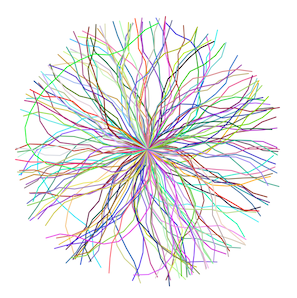• ## Turtle随机生成图像

千次阅读 2016-07-09 23:37:20
利用turtle随机生成图形
随机生成图形

import turtle
import random

def drawShape(sides, length):
angle = 360.0 / sides
for side in range(sides):
turtle.forward(length)
turtle.right(angle)

def moveTurtle(x, y):
turtle.penup()      //提笔，不会显示线
turtle.goto(x, y)
turtle.pendown()

def drawSquare(length):
drawShape(4, length)

def drawTriangle(length):
drawShape(3, length)

def drawCircle(length):
drawShape(360, length)

def drawRandom():
x = random.randrange(-200, 200)
y = random.randrange(-200, 200)
length = random.randrange(75)
shape = random.randrange(1, 4)
print shape

moveTurtle(x, y)

if shape == 1:
drawSquare(length)
elif shape == 2:
drawTriangle(length)
elif shape == 3:
length = length % 4
drawCircle(length)

for shape in range(100):
drawRandom()

turtle.done()

展开全文python
• while turtle.distance(0, 0) (-MAX_ANGLE, MAX_ANGLE + 1) turtle.right(angle) turtle.forward(pieceLength) def jumpToCenter(turtle): turtle.penup() turtle.home() turtle.pendown() def randomColor(turtle):...
Any idea why these lines aren't in color?这很简单，因为你从来没有真正调用过randomColor()Rather than using for i in range(pieces) to draw lines that are basedon the number of segments, how can I use a while loop to draw jaggedlines that each have a distance of 250?在这里，我们可以利用turtle未被充分利用的.distance()方法来告诉我们离中心有多远。这是直线距离，而不是路径行驶距离，它似乎与您的目标图示相符：from random import randrangefrom turtle import Turtle, ScreenMAX_ANGLE = 30MAX_DISTANCE = 250def jaggedLine(turtle, pieceLength):randomColor(turtle)while turtle.distance(0, 0) < MAX_DISTANCE:angle = randrange(-MAX_ANGLE, MAX_ANGLE + 1)turtle.right(angle)turtle.forward(pieceLength)def jumpToCenter(turtle):turtle.penup()turtle.home()turtle.pendown()def randomColor(turtle):r = randrange(255)  # red component of colorg = randrange(255)  # green componentb = randrange(255)  # blue componentturtle.pencolor(r, g, b)def main():s = Screen()s.colormode(255)t = Turtle()t.pensize(2)t.speed('fastest')  # because I have no patiencefor angle in range(0, 360, 2):jumpToCenter(t)t.setheading(angle)jaggedLine(t, 30)t.hideturtle()s.mainloop()if __name__ == "__main__":main()输出展开全文• 1.引入模板(1)import语句(以turtle模板为例)每个import语句只能导入一个模块，一般按照标准库、扩展库、自定义库顺序导入想使用 Python 源文件，只需在另一个源文件里执行 import 语句，语法如下：importmodule1[,...
1.引入模板(1)import语句(以turtle模板为例)每个import语句只能导入一个模块，一般按照标准库、扩展库、自定义库顺序导入想使用 Python 源文件，只需在另一个源文件里执行 import 语句，语法如下：importmodule1[,module2[,...moduleN]import math                    #导入标准库mathmath.sin(0.5)                  #求0.5(单位是弧度)的正弦import random                  #导入标准库randomn = random.random()            #获得[0,1) 内的随机小数print(n)n = random.randint(1,100)      #获得[1,100]区间上的随机整数print(n)n = random.randrange(1, 100)   #返回[1, 100)区间中的随机整数print(n)#使用turtle在屏幕上绘制图形turtle.pendown()  移动的时候绘制turtle.penup()      移动的时候不绘制turtle.pensize()    将线的粗细设定为指定宽度二：Turtle运动的方向turtle.forward(d)    移动方向前移指定距离turtle.backward(d) 移动方向反向移动指定距离turtle.left(angle)     左转指定角度turtle.right(angle)   右转指定角度turrle.goto(x,y)       移动到一个绝对位置turtle.setx(x)          将x坐标移动到指定位置turtle.sety(y)           将y坐标移动到指定位置turtle.setheading(angle)    将移动方向设置指定角度(0-东，90-北，180-西，270-南)turtle.home()                    移动到起点(0,0)向东turtle.circle(r,ext,step)       指定半径，范围和阶数的圆turtle.dot(diameter,color)    绘制一个指定直径和颜色的圆turtle.undo()                      取消最后一个操作turtle.speed(s)                   设置turtle的移动速度#运行绘图模板import turtle#画笔尺寸turtle.pensize(4)#画笔颜色turtle.pencolor('red')#前进距离turtle.forward(400)#前进方向turtle.right(135)turtle.forward(400)turtle.right(135)turtle.forward(400)turtle.right(135)turtle.forward(400)turtle.right(135)turtle.forward(400)turtle.right(135)turtle.forward(400)turtle.right(135)turtle.forward(400)turtle.right(135)turtle.forward(400)#turtle.mainloop()(2)from … import 语句Python 的 from 语句让你从模块中导入一个指定的部分到当前命名空间中，语法如下：frommodname importname1[,name2[,...nameN]]#from 模块名 import 对象名[ as 别名]from math import sinfrom math import cosprint(sin(30))print(cos(30))from math import sin as sprint(s(30))from … import * 语句把一个模块的所有内容全都导入到当前的命名空间也是可行的，只需使用如下声明：frommodname import*#import *from math import *print(sin(3))print(tan(1))
展开全文• /usr/bin/env python# coding=utf-8# 画一棵樱花import turtleimport randomfrom turtle import *from time import sleep# 画樱花的躯干(60,t)def tree(branchLen,t):sleep(0.0005)if branchLen >3:if 8<...
废话不多说了，直接上代码吧！#!/usr/bin/env python# coding=utf-8# 画一棵樱花import turtleimport randomfrom turtle import *from time import sleep# 画樱花的躯干(60,t)def tree(branchLen,t):sleep(0.0005)if branchLen >3:if 8<= branchLen <=12:if random.randint(0,2) == 0:t.color('snow') # 白else:t.color('lightcoral') # 淡珊瑚色t.pensize(branchLen / 3)elif branchLen <8:if random.randint(0,1) == 0:t.color('snow')else:t.color('lightcoral') # 淡珊瑚色t.pensize(branchLen / 2)else:t.color('sienna') # 赭(zhě)色t.pensize(branchLen / 10) # 6t.forward(branchLen)a = 1.5 * random.random()t.right(20*a)b = 1.5 * random.random()tree(branchLen-10*b, t)t.left(40*a)tree(branchLen-10*b, t)t.right(20*a)t.up()t.backward(branchLen)t.down()# 掉落的花瓣def petal(m, t):for i in range(m):a = 200 - 400 * random.random()b = 10 - 20 * random.random()t.up()t.forward(b)t.left(90)t.forward(a)t.down()t.color('lightcoral') # 淡珊瑚色t.circle(1)t.up()t.backward(a)t.right(90)t.backward(b)def main():# 绘图区域t = turtle.Turtle()# 画布大小w = turtle.Screen()t.hideturtle() # 隐藏画笔getscreen().tracer(5,0)w.screensize(bg='wheat') # wheat小麦t.left(90)t.up()t.backward(150)t.down()t.color('sienna')# 画樱花的躯干tree(60,t)# 掉落的花瓣petal(200, t)w.exitonclick()main()以上这篇python3实现用turtle模块画一棵随机樱花树就是小编分享给大家的全部内容了，希望能给大家一个参考您可能感兴趣的文章:
展开全文• 最近接触到 Python的一个绘图模块 Turtle，学习以后，发现这是一个很有趣的模块。我们可以利用这个模块进行画图，甚至做一些怀旧的小游戏。这个模块的文档链接如下。...比如说，我打算生成一个随机的线路...
• 通过Python随机生成坐标绘制心形❤ #绘制心形的方法 def drawCircle(): pencolor("red") fillcolor("pink") begin_fill() pendown() left(135) circle(20,180) forward(40) left(90) forward(40) ...Python random
• 使用turtle画树，源代码转载的地方忘记了，我自己编译运行后，修改了部分代码， 使用turtle画一颗树，树枝桠数量随机生成，添加花瓣特效。
• 用Python turtle经过简单的重复与...代码绘图案例1下图就是用同一代码模版生成的(代码见文末)。代码绘图案例2from turtle import *from random import *Screen().bgcolor("yellow")colormode(255)#设置颜色模式spe...
• 使用turtle生成随机生成不同颜色的圆圈 import turtle import random #随机生成RBG颜色代码（参考来自于CSDN） def randomcolor(): colorArr=[‘1’,‘2’,‘3’,‘4’,‘5’,‘6’,‘7’,‘8’,‘9’,‘A’,‘B’,...Python
• 对于我的课，我的任务是编程一个海龟函数，这个函数是用户...到目前为止，我有这个：from turtle import *from random import randrangeFRAMES_PER_SECOND = 10mirrorTurtle = Turtle()def turnRight():global tu...
• 前面已经接触了用turtle模块画图，并且了解了自定义函数的写法，那么根据前面的知识，我们接触下random模块；首先引入 import random；random.randrange(x):用于生成0-x之间的随机数；random.randrange(x,y):用于...
• 前面已经接触了用turtle模块画图，并且了解了自定义函数的写法，那么根据前面的知识，我们接触下random模块； 首先引入 import random； random.randrange(x):用于生成0-x之间的随机数； random.randrange(x,y):...
• 【其它】一个整数,它加上100后是一个完全平方数...【单选题】哪个选项不能正确引用turtle库进而使用setup()函数?【单选题】下面不属于Python保留字的是:【其它】若x、y、z均为非负偶整数,编写程序列出下列方程组的所...
• 如上图所示，数据集由一系列随机生成的图像组成，每幅图像包含了40~50个大小相等的“T"图案与一个”L"图案，它们在图像中的位置、方向都是无规则的，因此可以作为目标检测、语义分割等任务的数据集。 二、编程思路 ...Python Dataset
• # SnowView.py import turtle import random # -------绘制雪花--------------- ... # 雪花的RGB颜色参数，随机生成一个【0.0， 1.0）之间的数 r, g, b = random.random(), random.random(), random.random()
• turtle图形艺术效果中隐含着很多随即元素，如随机颜色，尺寸，位置和数量等。因此在图形艺术绘制中需要引入随机函数库random。常用randint（）函数生成指定范围内的随机数。 本实例选择“雪景”作为编程目标。 ...实例 python
• 最近学习了随机生成迷宫的算法， 分享学习经验以及碰到的问题点。目前学习两个算法 生成随机地图， 深度优先算法和广度优先算法来生成迷宫。比较下他们的不同点。 在程序中，我们用数组M表示所有的单元格子的属算法 python
• 问题：turtle.click()鼠标点击的是一个坐标点，很难点到，... #随机生成0~10的整数赋值给x/y x = random.randint(1,1000) y = random.randint(1,1000) t.goto(x,y) turtle.onclick(go_home,btn=1,add=True)onclick python random
• 作为一个世界上非著名非专业的画家，当然得让大家先欣赏下我的...这棵樱花树，我们使用了python的turtle模块，利用一些数学函数，将随机的画出不同的树枝以及飘落的花瓣，我们每次运行都会生成一棵相对独一无二的...
• 打字小游戏原理根据字母的unicode编码 生成相应的随机数 在把随机数转换成字母 关键就是下面两...然后根据键盘按下事件 判断按下的键(keyCode)和随机生成字母(zmcode)有没有相等就可以知道你输入的字母有没有正确,错...
• #生成随机颜色方法（16进制） `def randomcolor(): colorArr = [‘0’,‘1’,‘2’,‘3’,‘4’,‘5’,‘6’,‘7’,‘8’,‘9’,‘A’,‘B’,‘C’,‘D’,‘E’,‘F’] color = “” for i in range(6): color +...python
• 勾股树画法（角度随机）：此勾股树的画法采取角度随机的方式进行，树的形状与现实树比较接近，每次运行生成的树形状都不一样 先上图： 代码如下： ‘’’ 勾股树画法（角度随机）： 此勾股树的画法采取角度随机的...
• 运用turtle生成的石头剪刀布游戏，实现人机对打（其中计算机是随机生成），具有很好的趣味性
• 我有随机生成正方形的代码..但是我在用原色随机填充正方形方面遇到麻烦吗？有谁知道该如何解决？这是我的代码：import turtleimport randomturtle.screensize(1000,1000)turtle.setworldcoordinates(-500,-500,500,...
• 再结合随机函数生成任意的一棵树， 樱花树主要组成部分有树干和花瓣以及飘落的花瓣构成。 import turtle import random import time 需要以上模块 1.画樱花的躯干 绘画的树干使用了 Python 中的随机函数， 这样每次...python
• 常用randint()函数生成指定范围内的随机数，例如randint(-350,350)生成-350～350的随机整数 选择雪景作为编程目标 雪景图形艺术背景为黑色，分为上下两个区域，上方是漫天彩色雪花，下方是由远及近的.python 数据可视化
• python应用实例：雪景绘图 turtle图形艺术，指利用... turtle图形艺术效果中隐含着很多随机元素,如随机颜色、尺寸、位置 和数量等。在图形艺术绘制中需要引入随机函数random。常用randint() 函数,生成指定范围...python random